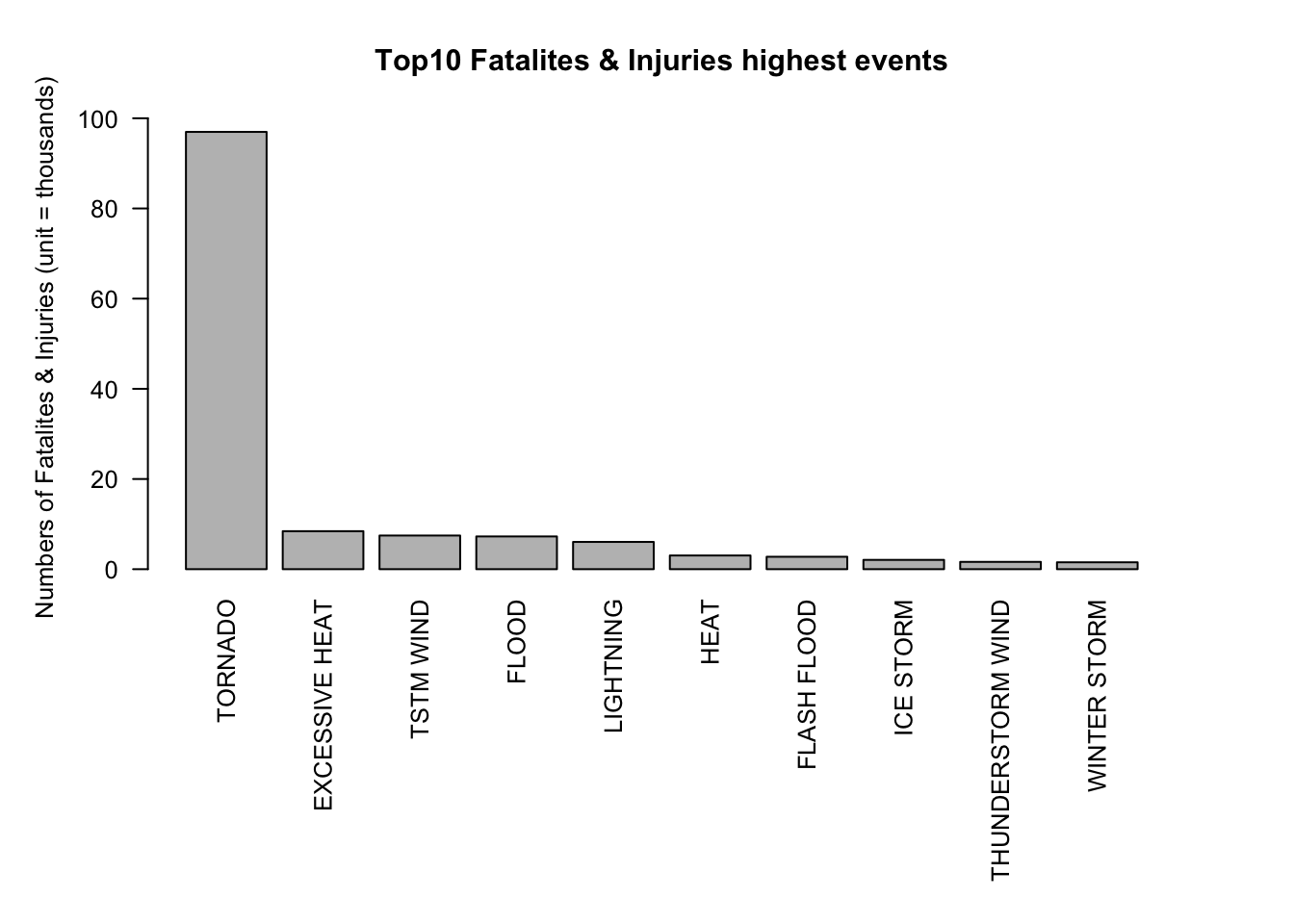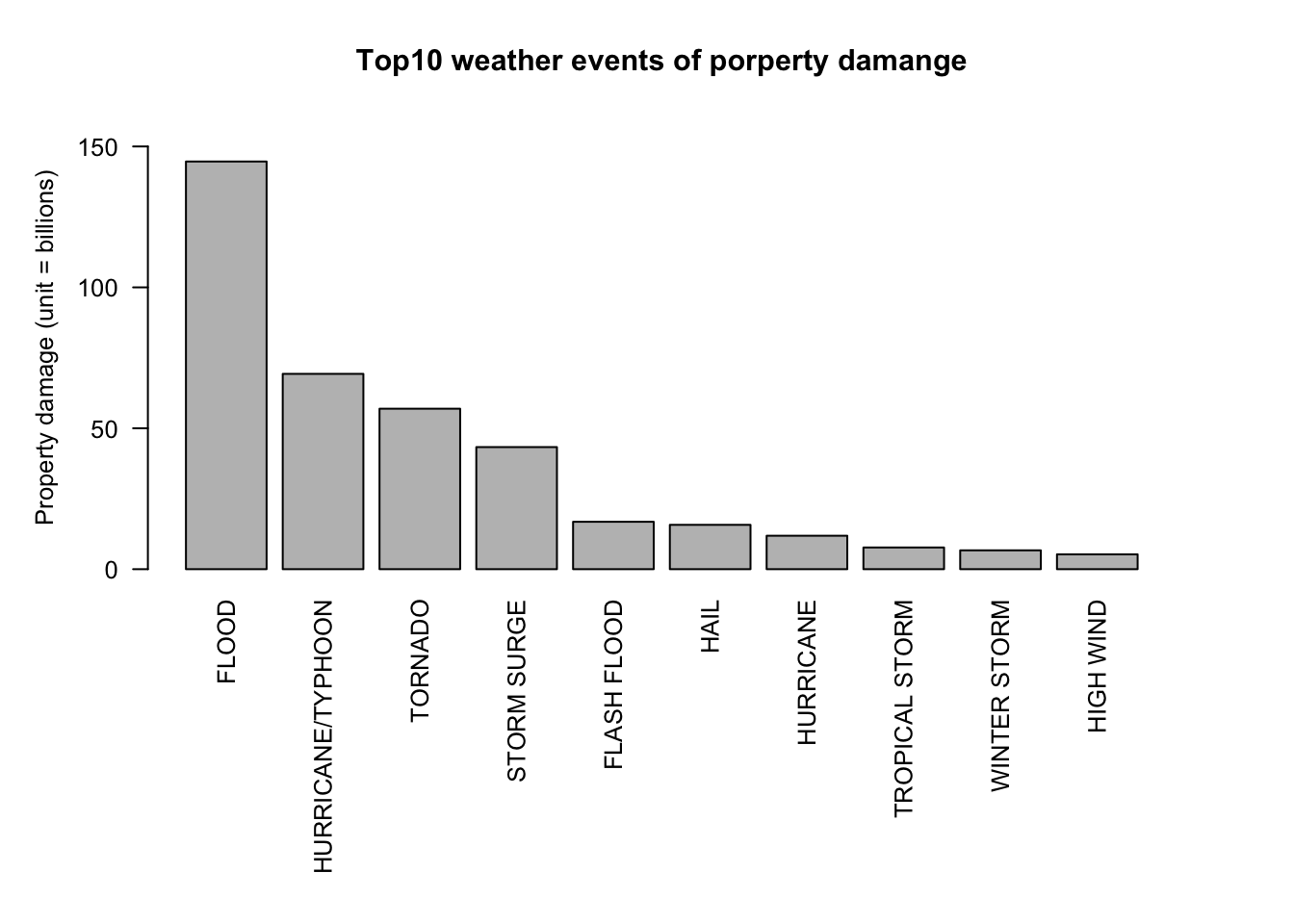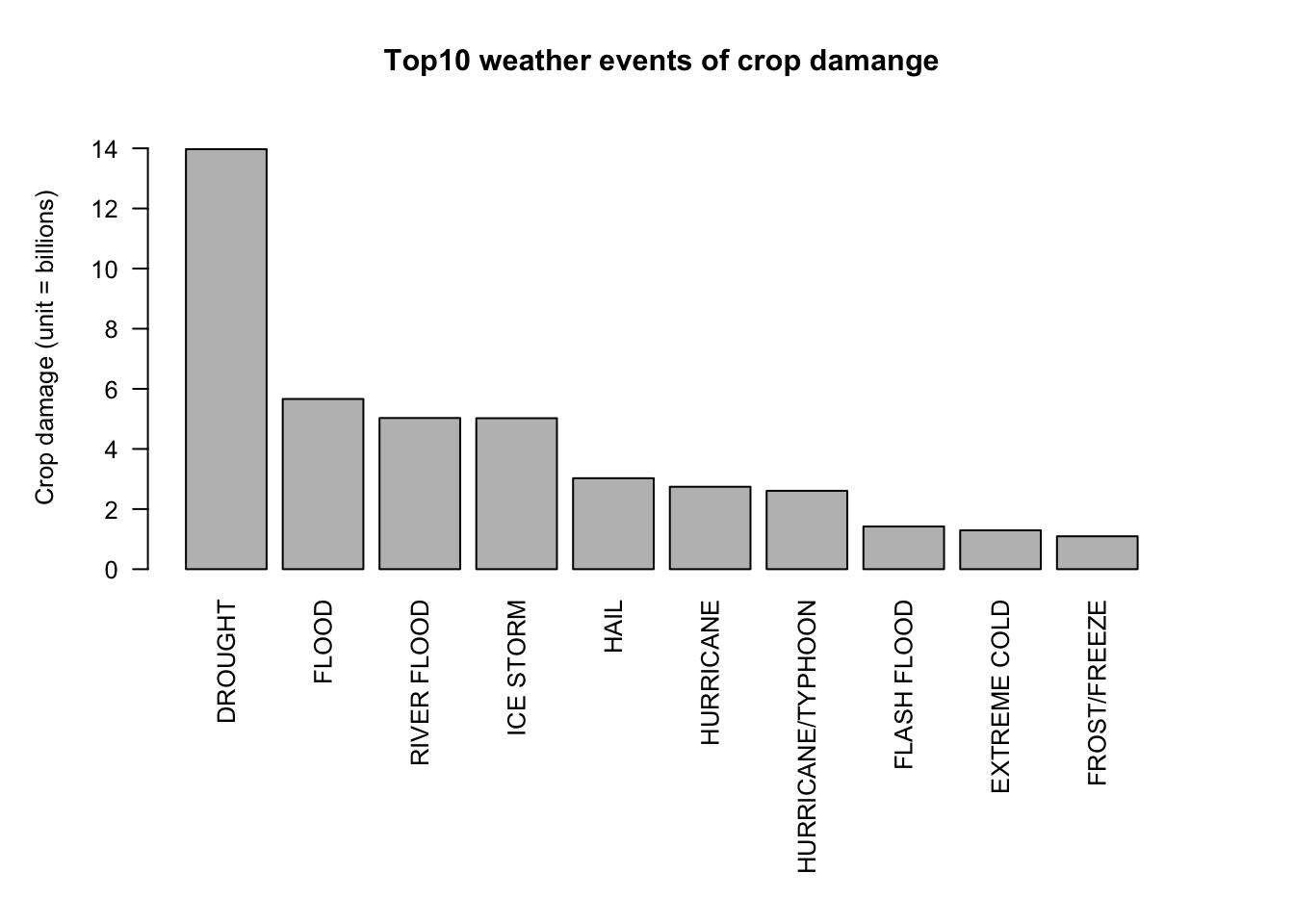## 1. Introduction

Extreme weather events cause huge damage to the environment and humans.This analysis involves exploring the U.S. National Oceanic and Atmospheric Administration’s (NOAA) storm database. This database tracks extreme weather events across the United States from 1950 to 2011, and counts the casualties, property damage, and crop damage caused by extreme weather events.

In this analysis, we will try to observe and analyze the impact of extreme weather events on various indicators.

## 2. synopsis

This analysis will provide the answers of two questions as below:

* Across the United States, which types of events are most harmful with respect to population health?

* Across the United States, which types of events have the greatest economic consequences?

The database start in the year 1950 and end in November 2011 and more information about variables definitions as follows:

## 3. Data Processing

The data set which I processed is download from Couersera Reproducible Research course.
https://d396qusza40orc.cloudfront.net/repdata%2Fdata%2FStormData.csv.bz2

First, I download dataset and extract it as a dataframe named “df”.

dataURL <- "https://d396qusza40orc.cloudfront.net/repdata%2Fdata%2FStormData.csv.bz2"

if(!file.exists("./repdata-data-StormData.csv.bz2") ) {
}
df <- read.csv(bzfile("./repdata-data-StormData.csv.bz2"), header = TRUE)

Next,check the dataset with dim( ) & str( ).

dim(df)
##  902297     37
str(df)
## 'data.frame':    902297 obs. of  37 variables:
##  $STATE__ : num 1 1 1 1 1 1 1 1 1 1 ... ##$ BGN_DATE  : chr  "4/18/1950 0:00:00" "4/18/1950 0:00:00" "2/20/1951 0:00:00" "6/8/1951 0:00:00" ...
##  $BGN_TIME : chr "0130" "0145" "1600" "0900" ... ##$ TIME_ZONE : chr  "CST" "CST" "CST" "CST" ...
##  $COUNTY : num 97 3 57 89 43 77 9 123 125 57 ... ##$ COUNTYNAME: chr  "MOBILE" "BALDWIN" "FAYETTE" "MADISON" ...
##  $STATE : chr "AL" "AL" "AL" "AL" ... ##$ EVTYPE    : chr  "TORNADO" "TORNADO" "TORNADO" "TORNADO" ...
##  $BGN_RANGE : num 0 0 0 0 0 0 0 0 0 0 ... ##$ BGN_AZI   : chr  "" "" "" "" ...
##  $BGN_LOCATI: chr "" "" "" "" ... ##$ END_DATE  : chr  "" "" "" "" ...
##  $END_TIME : chr "" "" "" "" ... ##$ COUNTY_END: num  0 0 0 0 0 0 0 0 0 0 ...
##  $COUNTYENDN: logi NA NA NA NA NA NA ... ##$ END_RANGE : num  0 0 0 0 0 0 0 0 0 0 ...
##  $END_AZI : chr "" "" "" "" ... ##$ END_LOCATI: chr  "" "" "" "" ...
##  $LENGTH : num 14 2 0.1 0 0 1.5 1.5 0 3.3 2.3 ... ##$ WIDTH     : num  100 150 123 100 150 177 33 33 100 100 ...
##  $F : int 3 2 2 2 2 2 2 1 3 3 ... ##$ MAG       : num  0 0 0 0 0 0 0 0 0 0 ...
##  $FATALITIES: num 0 0 0 0 0 0 0 0 1 0 ... ##$ INJURIES  : num  15 0 2 2 2 6 1 0 14 0 ...
##  $PROPDMG : num 25 2.5 25 2.5 2.5 2.5 2.5 2.5 25 25 ... ##$ PROPDMGEXP: chr  "K" "K" "K" "K" ...
##  $CROPDMG : num 0 0 0 0 0 0 0 0 0 0 ... ##$ CROPDMGEXP: chr  "" "" "" "" ...
##  $WFO : chr "" "" "" "" ... ##$ STATEOFFIC: chr  "" "" "" "" ...
##  $ZONENAMES : chr "" "" "" "" ... ##$ LATITUDE  : num  3040 3042 3340 3458 3412 ...
##  $LONGITUDE : num 8812 8755 8742 8626 8642 ... ##$ LATITUDE_E: num  3051 0 0 0 0 ...
##  $LONGITUDE_: num 8806 0 0 0 0 ... ##$ REMARKS   : chr  "" "" "" "" ...
##  $REFNUM : num 1 2 3 4 5 6 7 8 9 10 ... This data is huge,there are 902297 observations with 37 variables in the file and some variables format need to be adjusted. Only a subset is required for the analysis and subset include 7 variables are as follows: • EVTYPE : weather type. • FATALITIES, INJURIES: the number of fatalities/injuries due to weather events. • PROPDMG, PROPDMGEXP: estimate of property damage value. • PCROPDMG, CROPDMGEXP: estimate of crop damage value. df <- subset(df, select = c("EVTYPE","FATALITIES","INJURIES","PROPDMG","PROPDMGEXP","CROPDMG","CROPDMGEXP")) The economic losses are estimated from these variables: “PROPDMG/PROPDMGEXP” and “CROPDMG/CROPDMGEXP”. I found that there are some characters in variable columns, that can’t calculated, so I transform those into a proper format. unique(df$PROPDMGEXP)
##   "K" "M" ""  "B" "m" "+" "0" "5" "6" "?" "4" "2" "3" "h" "7" "H" "-" "1" "8"

Consider numbers & K|M|B|H as dollars, other definitions as 0.

df$PROPDMGEXP <- as.character(df$PROPDMGEXP)
df$PROPDMGEXP[!grep("K|M|B|5|6|4|2|3|h|7|1|8", df$PROPDMGEXP, ignore.case = TRUE)] <- 0
df$PROPDMGEXP[grep("K", df$PROPDMGEXP, ignore.case = TRUE)] <- "3"
df$PROPDMGEXP[grep("M", df$PROPDMGEXP, ignore.case = TRUE)] <- "6"
df$PROPDMGEXP[grep("B", df$PROPDMGEXP, ignore.case = TRUE)] <- "9"
df$PROPDMGEXP[grep("h", df$PROPDMGEXP, ignore.case = TRUE)] <- "2"

A new variable created to estimate of property damage value.

df$PROPDMGEXP <- as.numeric(as.character(df$PROPDMGEXP))
df$PROPDMG.total <- df$PROPDMG * 10^df$PROPDMGEXP/1000000000 # unit = billions Than, process crop damage data in the same ways. unique(df$CROPDMGEXP)
##  ""  "M" "K" "m" "B" "?" "0" "k" "2"
df$CROPDMGEXP[!grep("K|M|B|2", df$CROPDMGEXP, ignore.case = TRUE)] <- 0
df$CROPDMGEXP[grep("K", df$CROPDMGEXP, ignore.case = TRUE)] <- "3"
df$CROPDMGEXP[grep("M", df$CROPDMGEXP, ignore.case = TRUE)] <- "6"
df$CROPDMGEXP[grep("B", df$CROPDMGEXP, ignore.case = TRUE)] <- "9"
df$CROPDMGEXP <- as.numeric(as.character(df$CROPDMGEXP))
df$CROPDMG.Total <- df$CROPDMG * 10^df$CROPDMGEXP/1000000000 # unit = billions ## 4. Results ### 4.1 The first question: Across the United States, which types of events are most harmful with respect to population health? To answer this question, let’s observe the number of fatality/injured people by weather events. fata <- aggregate(FATALITIES~EVTYPE, data=df, sum) #create a subset for analysis fata <- fata[order(-fata$FATALITIES),]
head(fata)
##             EVTYPE FATALITIES
## 130 EXCESSIVE HEAT       1903
## 153    FLASH FLOOD        978
## 275           HEAT        937
## 464      LIGHTNING        816
## 856      TSTM WIND        504

In result, the first and second items are bigger than others. Let’s check injured data.

inju <- aggregate(INJURIES~EVTYPE, data=df, sum) #create a subset for analysis
inju <- inju[order(-inju$INJURIES),] head(inju) ## EVTYPE INJURIES ## 834 TORNADO 91346 ## 856 TSTM WIND 6957 ## 170 FLOOD 6789 ## 130 EXCESSIVE HEAT 6525 ## 464 LIGHTNING 5230 ## 275 HEAT 2100 In injured data, the first item is more huge than the others item ,otherwise the biggest items from fatality and injured data are caused by TORNADO. According to the observations, I merge the number of fatalities and injured into a modified subset. library(dplyr) Q1 <- df %>% select(EVTYPE,FATALITIES,INJURIES)%>% group_by(EVTYPE)%>% summarise( FATsum = sum(FATALITIES)/1000, INJsum = sum(INJURIES)/1000, Total = (FATsum + INJsum)) %>% arrange(desc(Total)) The variable “Total” is the sum of fatalities and injured data.Next, I arrange and select the Top 10 and plot it. Q1.plot <- Q1[1:10,] par(mar=c(12,5,4,4), cex=0.8) barplot(Q1.plot$Total, names.arg = Q1.plot$EVTYPE, las =2, ylim = c(0, 100), main = "Top10 Fatalites & Injuries highest events", ylab = "Numbers of Fatalites & Injuries (unit = thousands) ")Answer of first question, TORNADO is the most harmful to population. ### 4.2 The second question: Across the United States, which types of events have the greatest economic consequences? First of all, we first observe the top 10 highest property losses by weather events and plot it. df.PROPDMG <- aggregate(PROPDMG.total~EVTYPE, data=df, sum) df.PROPDMG.top10 <- df.PROPDMG[order(-df.PROPDMG$PROPDMG.total),][1:10,]
head(df.PROPDMG.top10)
##                EVTYPE PROPDMG.total
## 62              FLOOD     144.65771
## 179 HURRICANE/TYPHOON      69.30584
## 281       STORM SURGE      43.32354
## 50        FLASH FLOOD      16.82267
## 103              HAIL      15.73527
par(mar=c(12,5,4,4), cex=0.8)
barplot(df.PROPDMG.top10$PROPDMG.total, names = df.PROPDMG.top10$EVTYPE,
las=2,
ylim = c(0, 160),
main = "Top10 weather events of porperty damange",
ylab = "Property damage (unit = billions) ")Next step, we observe the top 10 highest crop losses by weather events and plot it.

df.CROPDMG <- aggregate(CROPDMG.Total~EVTYPE, data=df, sum)
df.CROPDMG.top10 <- df.CROPDMG[order(-df.CROPDMG$CROPDMG.Total),][1:10,] par(mar=c(12,5,4,4), cex=0.8) barplot(df.CROPDMG.top10$CROPDMG.Total,
names = df.CROPDMG.top10\$EVTYPE,
las=2,
ylim = c(0, 15),
main = "Top10 weather events of crop damange",
ylab = "Crop damage (unit = billions) ")